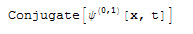## The Problem

Mathematica derives expressions with complex variables and functions by using the chain rule, as shown in the following example:It is unclear, what the derivation of the Conjugate function means anyway.

In applications of the Quantum Mechanics one often uses the wave function Ψ, having itself complex values but depending from real parameters like the time t or the location x.

For this application scenario the following solution would make sense:## The Goal

My goal was to create an own function d  (as replacement for D) with the following properties:

• it shall support the same parameters as D
• for Conjugate expressions the former rule becomes effective meaning derivations of Conjugates will become Conjugates of the derivation of the parameter expression of the Conjugates
• d performs an expression analysis for this objective and applies the rule described

## Program and Way of Functioning

The program displayed below runs under Mathematica 7+.

### Program Code

``````(* Substitute of the built in function D *)
d[ex_,vars__]:=
Module[{res,lis={},prepareVars},
SetAttributes[expSubstitute,HoldRest];
SetAttributes[replaceConjugate,HoldRest];
prepareVars[vars2_]:=Module[{},
If[Depth[vars2]<=2,
vars2,
Map[#[]&,vars2]
]
];
res=expSubstitute[ex,{Sequence[prepareVars[{vars}]]},replaceConjugate,lis];         (* transformation *)
res=D[res,vars]; (* perform the derivation *)
res=expSubstitute[res,{vars},replacePlaceHolder,lis];                               (* back transformation *)
res
](* Common Substitution Function *)
expSubstitute[exp_,{vars__},getReplacement_:Function[{ex,lis,vars},ex],lis_,level_:0]:=
Module[{return,i},
If[
AtomQ[exp],
Return exp;
];
For[
i=2,
i<=Length[return],
i=i+1,
If[!AtomQ[return[[i]]],
return[[i]]=expSubstitute[return[[i]],{vars},getReplacement,lis,level+1];
];
];
return=getReplacement[return,lis,{vars}];
return=Apply[First[return],Drop[return,1]];
return
](* Replacement of Conjugate with Placeholder *)
replaceConjugate[x_,lis_,{vars__}]:=
Module[{},
If[SameQ[x[],Conjugate],
AppendTo[lis,x[]];
{\[Psi][Length[lis]],vars},
x
]
](* Replacement of Placeholder back to Original Expression *)
replacePlaceHolder[x_,lis_,{vars__}]:=
{Conjugate,D[lis[[m1]],vars]},
{Conjugate,lis[[m1]]},
True,
x
]
]``````

### Composition and Operation

This Mathematica module consists of 4 functions:

 d the derivation function itself expSubstitute analyses an expression and performs a substitution replaceConjugate replaces Conjugate expressions inside a composite expression with a placeholder replacePlaceHolder replaces the placeholder back as being explained below

The funktion d works as explained below:

• first all Conjugate functions are replaced by placeholders
• for this purpose the function expSubstitute is called with the parameter function replaceConjugate as argument
• subsequently the built-in function D is applied
• finally expSubstitute is called again with the parameter function replacePlaceHolder as argument
• by doing this the placeholder is translated back in Conjugate expressions
• derivations of the placeholder now become Conjugates of derivations of the arguments of the original Conjugates